Question

Three fair six-sided dice are rolled. a) What is the probability of seeing {1, 3, 6}?

b) What is the probability of seeing {1, 4, 4}?

c) What is the probability of seeing {2, 2, 2} ?

d) What is the probability of seeing at least one 6?

e) What is the probability that the sum of all three dice is 16?

f) What is the probability of seeing exactly two even numbers?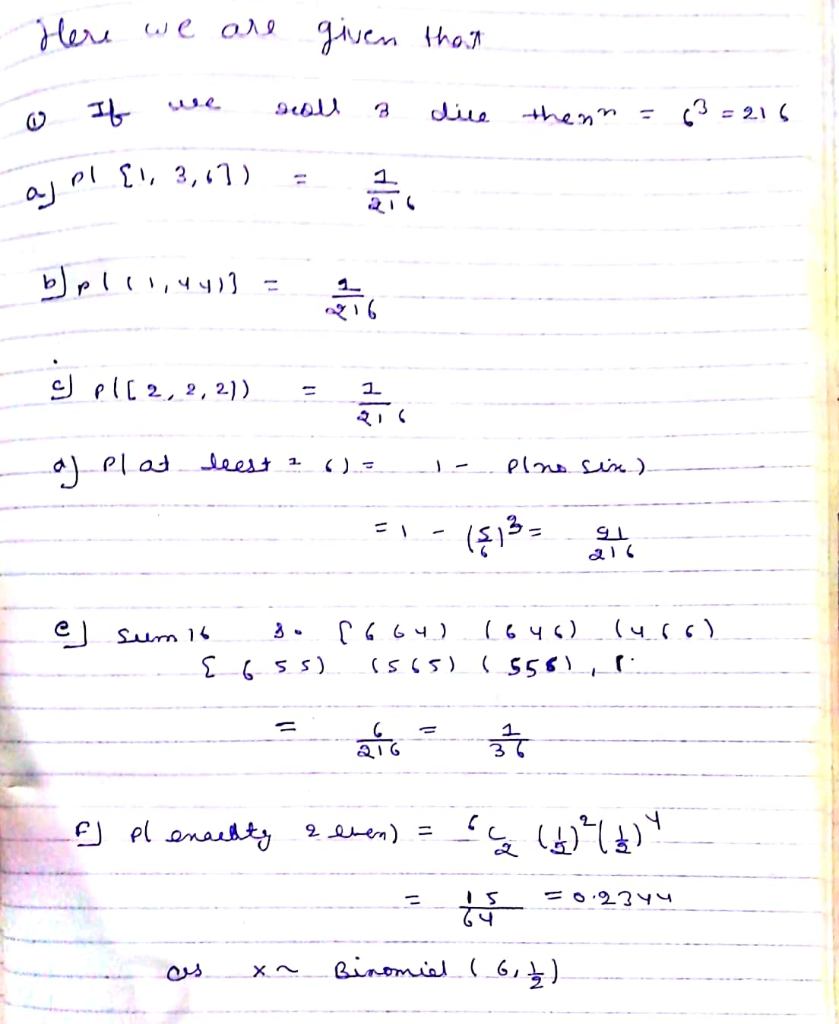#### Earn Coins

Coins can be redeemed for fabulous gifts.

Similar Homework Help Questions
• ### Two fair six-sided dice are rolled. What is the probability that one die shows exactly three...

Two fair six-sided dice are rolled. What is the probability that one die shows exactly three more than the other die (for example, rolling a 1 and 4, or rolling a 6 and a 3)

• ### Three six-sided fair dice are rolled. The six sides are numbered 1,2,3,4,5,6. Let A be the...

Three six-sided fair dice are rolled. The six sides are numbered 1,2,3,4,5,6. Let A be the event that the first die shows an even number, let B be the event that the second die shows an even number, and let C be the event that the third die shows an even number. Express each of the following events in terms of the named events described above: 1) the event that all three dice show even numbers 2) the event that...

• ### 1. A blue fair 6-sided dice and a red fair 6-sided dice are rolled at the...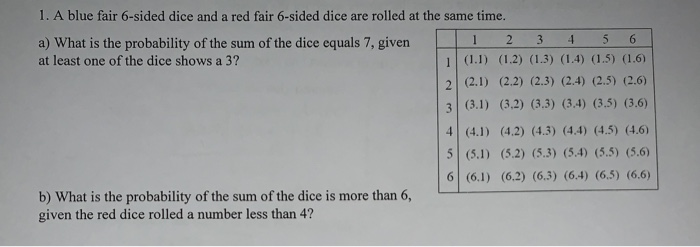1. A blue fair 6-sided dice and a red fair 6-sided dice are rolled at the same time. a) What is the probability of the sum of the dice equals 7, given 1 2 3 4 5 6 at least one of the dice shows a 3? 1 (1.1) (1.2) (1.3) (1.4) (1.5) (1.6) 2 (2.1) (2.2) (2.3) (2.4) (2.5) (2.6) (3.1) (3.2) (3.3) (3.4) (3.5) (3.6) (4.1) (4.2) (4.3) (4.4) (4.5) (4.6) 5 (5.1) (5.2) (5.3) (5.4) (5.5) (5.6)...

• ### 6. A fair six sided die is rolled three times. Find the probability that () all...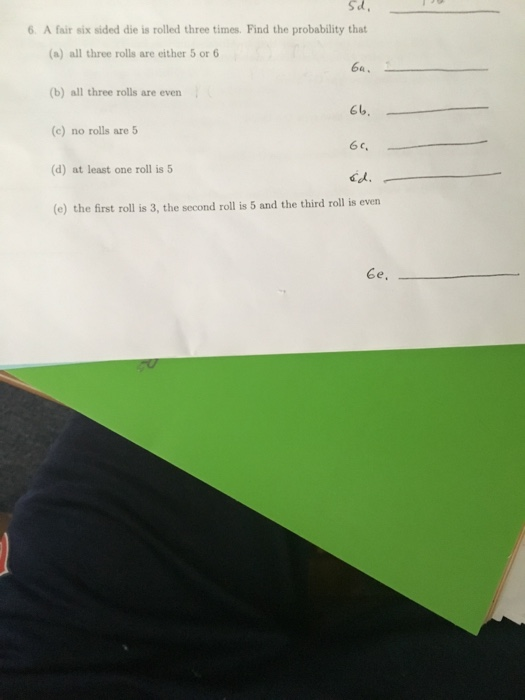6. A fair six sided die is rolled three times. Find the probability that () all three rolls are either 5 or 6 (6) all three rolls are even (c) no rolls are 5 (d) at least one roll is 5 (e) the first roll is 3, the second roll is 5 and the third roll is even

• ### Three six-faced fair dice are rolled. What is the probability that exactly two of the dice...

Three six-faced fair dice are rolled. What is the probability that exactly two of the dice have the face value of 6?

• ### If two six sided dice are rolled, what is the probability of rolling a 5 on...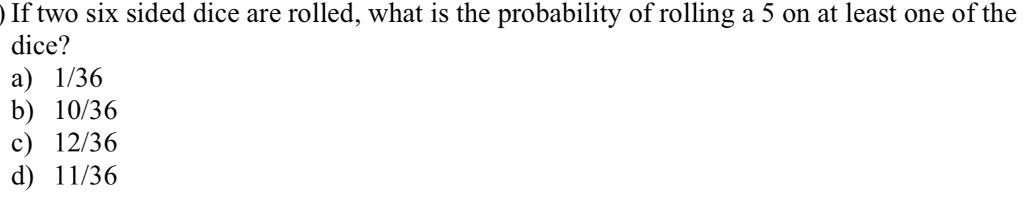If two six sided dice are rolled, what is the probability of rolling a 5 on at least one of the dice? a) I/36 b) 10/36 c) 12/36 d) 11/36

• ### Two six-sided dice are rolled. Determine the probability of the following events: a) The second dice...

Two six-sided dice are rolled. Determine the probability of the following events: a) The second dice shows the number two. b) The sum of dice is nine or more. c) None of the dice show a one. I would like a full solution for all of these three questions, if possible please.

• ### Conditional Probability Two fair dice are rolled: (a) Express the sample space S in set builder...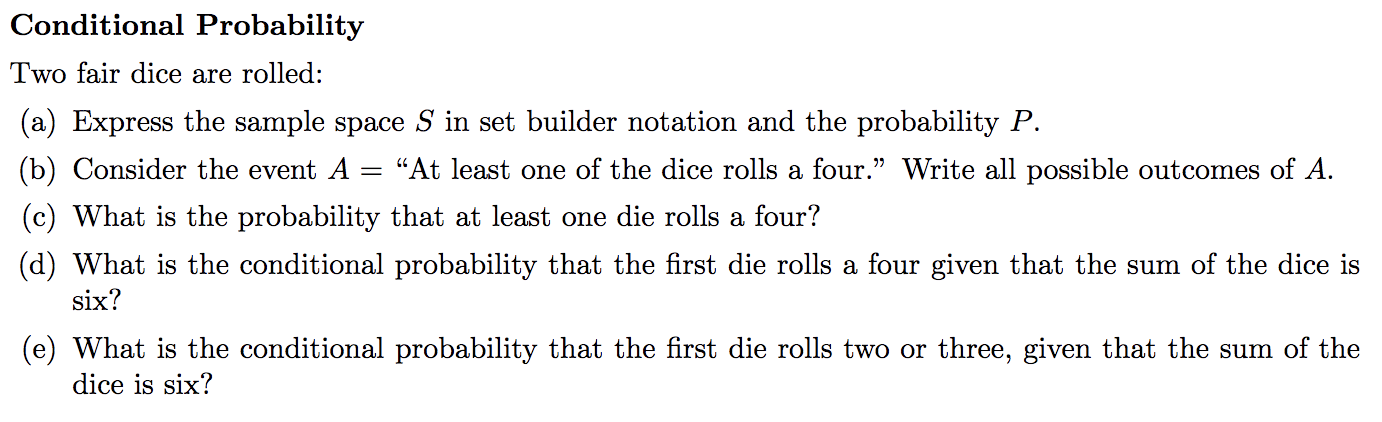Conditional Probability Two fair dice are rolled: (a) Express the sample space S in set builder notation and the probability P "At least one of the dice rolls a four." Write all possible outcomes of A (b) Consider the event A (c) What is the probability that at least one die rolls a four? (d) What is the conditional probability that the first die rolls a four given that the sum of the dice is six? (e) What is the...

• ### Suppose that 16 fair, 6-sided dice are rolled together (but independently). (a) What is the probability...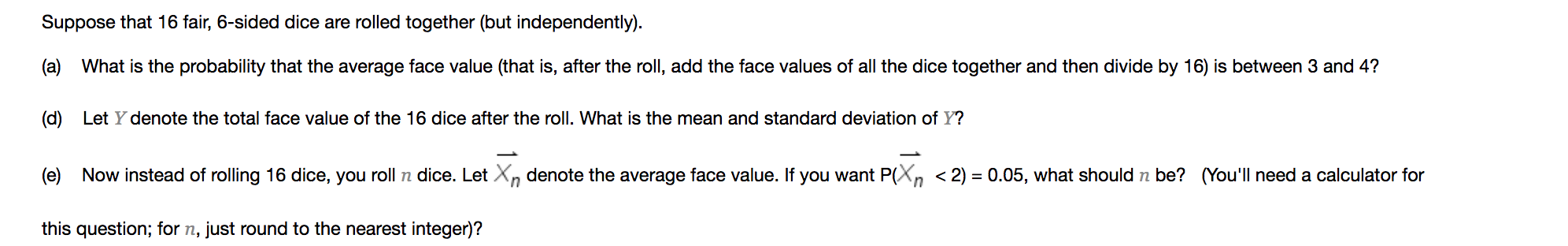Suppose that 16 fair, 6-sided dice are rolled together (but independently). (a) What is the probability that the average face value (that is, after the roll, add the face values of all the dice together and then divide by 16) is between 3 and 4? (d) Let Y denote the total face value of the 16 dice after the roll. What is the mean and standard deviation of Y? (e) Now instead of rolling 16 dice, you roll n dice....

• ### Problem #3: 5 fair 12-sided dice are rolled. (a) [3 marks] Find the conditional probability that...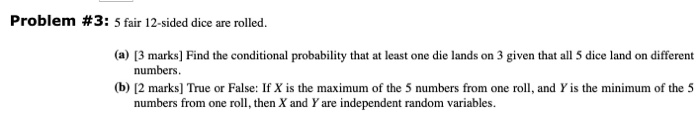Problem #3: 5 fair 12-sided dice are rolled. (a) [3 marks] Find the conditional probability that at least one die lands on 3 given that all 5 dice land on different numbers. 6) [2 marks] True or False: If X is the maximum of the 5 numbers from one roll, and Y is the minimum of the 5 numbers from one roll, then X and Y are independent random variables.